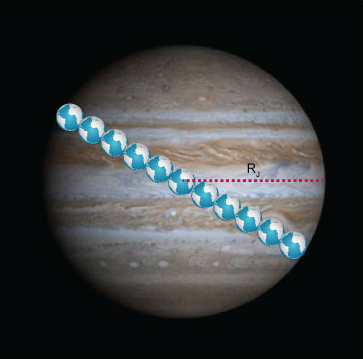An introduction to exoplanets

Start this free course now. Just create an account and sign in. Enrol and complete the course for a free statement of participation or digital badge if available.

Free course

# 4.3  Scaling radii and volumes

You learned that Jupiter is a factor of about 10 bigger than the Earth in size, meaning that the average radius of Jupiter is about 10 times the size of the radius of the Earth. In fact, it is 10.97 times as great to be more exact. This means that nearly 11 Earths could fit across Jupiter (Figure 9).Figure 9  The diameter of Jupiter is 11 times the diameter of Earth, hence the radius of Jupiter is 11 times the radius of Earth

For any shape, if the length is scaled up by a factor of 10, the volume will scale up by a factor of 1000. You compared cubes with sides differing by a factor of 10, and saw the volume differs by a factor of 1000. Similarly, the volume of a sphere with a radius of 10 m will be 1000 times as large as the volume of a sphere with radius 1 m. So, the volume of a sphere with a radius of 10 m will be 4.19 × 1000 = 4190 m3. It is easy to understand where this comes from: the radius is multiplied together three times during the calculation to get the volume. A factor of 10 multiplied by a factor of 10 multiplied by a factor of 10 will give a result 1000.

So, while Jupiter is a factor of over 10 larger than Earth in radius, it is over 1000 times larger in volume. This shows it is absolutely vital that you know exactly what you mean when you talk about how ‘big’ something is!

EXO_1